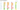# List of Sorting Algorithms

May 08, 2019This topic is one of the most common studied. When somebody started preparation of coding interviews. Sorting algorithms would in his top 2 topics. Even typical computer science graduate study sorting algorithms 3-4 times before they complete graduation.

## Why you should study Sorting Algorithms

• These are one of the most fundamental building block which helps in other complex algorithms.
• If you understand different tricks and basics around these algorithms, you can solve many-many more complex problems. Or, you can utilize the code somewhere.
• Most common techniques Divide and Conquer, covers few sorting algorithms. Which gives you more understanding of Divide and Conquer algorithms.
• In other computational algorithms, you must be using one of sorting algorithms either directly or indirectly.
• These are used in database queries

And, the list is endless.

## Which algorithm to study?

There are lot of sorting algorithms available, if you search in wikipedia. But, you should consider reading few of them. In below section, I will mention the important ones.

## Different Sorting Algorithms

There are lot of Sorting algorithms out there. I’ve categorized them as below:

### Most Basic Ones

Very basic ones. You should consider reading them at least once.

### Most Used and Optimized algorithms

Below algorithms must be on your tips.

• Merge Sort
• Quick Sort
• Heap Sort

### Other Algorithms

You should be familiar with these algorithms, although not much deep knowledge is required.

• Bucket Sort
• Counting Sort

### Time complexities of Sorting Algorithms

Algorithms Average(Expected) Running time Worst Running time
Insert Sort O(n^2) O(n^2)
Bubble Sort O(n^2) O(n^2)
Selection Sort O(n^2) O(n^2)
Quick Sort O(n log n) O(n^2)
Merge Sort O(n log n) O(n log n)
Heap Sort O(n log n) O(n log n)